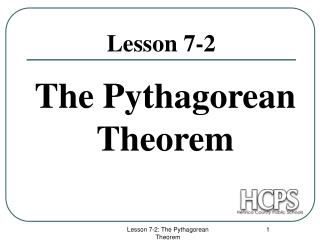DownloadDownload PresentationLesson 7-2

# Lesson 7-2

Download Presentation## Lesson 7-2

- - - - - - - - - - - - - - - - - - - - - - - - - - - E N D - - - - - - - - - - - - - - - - - - - - - - - - - - -
##### Presentation Transcript

1. The Pythagorean Theorem Lesson 7-2 Lesson 7-2: The Pythagorean Theorem

2. The Pythagorean Theorem Given any right triangle, A2 + B2 = C2 C A B Lesson 7-2: The Pythagorean Theorem

3. Example In the following figure if A = 3 and B = 4, Find C. A2 + B2 = C2 32 + 42 = C 2 9 + 16 = C2 5 = C C A B Lesson 7-2: The Pythagorean Theorem

4. You can verify the Pythagorean Theorem with the following: Given a piece of graph paper, make a right triangle. Then make squares of the right triangle. Then find the square’s areas. Lesson 7-2: The Pythagorean Theorem

5. A=8, B= 15, Find C A=7, B= 24, Find C A=9, B= 40, Find C A=10, B=24, Find C A =6, B=8, Find C Pythagorean Theorem : Examples C = 17 C = 25 C A C = 41 C = 26 B C = 10 Lesson 7-2: The Pythagorean Theorem

6. Finding the legs of a right triangle: In the following figure if B = 5 and C = 13, Find A. A2 + B2 = C2 A2 +52 = 132 A2 + 25 = 169 A2+25-25=169-25 A2 = 144 A = 12 C A B Lesson 7-2: The Pythagorean Theorem

7. 1) A=8, C =10 , Find B 2) A=15, C=17 , Find B 3) B =10, C=26 , Find A 4) A=15, B=20, Find C 5) A =12, C=16, Find B 6) B =5, C=10, Find A 7) A =6, B =8, Find C 8) A=11, C=21, Find B More Examples: B = 6 B = 8 A = 24 C = 25 C B = 10.6 A A = 8.7 C = 10 B = 17.9 B Lesson 7-2: The Pythagorean Theorem

8. Given the lengths of three sides, how do you know if you have a right triangle? Given A = 6, B=8, and C=10, describe the triangle. A2 + B2 = C2 62 +82 = 102 36 + 64 = 100 This is true, so you have a right triangle. C A B Lesson 7-2: The Pythagorean Theorem

9. Given A = 4, B = 5, and C =6, describe the triangle. A2 + B2 = C2 42 + 52 = 62 16 + 25 = 36 41 > 36, so we have an acute triangle. If A2 + B2 > C2, you have an acute triangle. A B C Lesson 7-2: The Pythagorean Theorem

10. Given A = 4, B = 6, and C =8, describe the triangle. A2 + B2 = C2 42 + 62 = 82 16 + 36 = 64 52 < 64, so we have an obtuse triangle. If A2 + B2 < C2, you have an obtuse triangle. A B C Lesson 7-2: The Pythagorean Theorem

11. 1) A=9, B=40, C=41 2) A=10, B=15, C=20 3) A=2, B=5, C=6 4) A=12, B=16, C=20 5) A=11, B=12, C=14 6) A=2, B=3, C=4 7) A=1, B=7, C=7 8) A=90, B=120, C=150 Describe the following triangles as acute, right, or obtuse right right obtuse right C acute A obtuse acute right B Lesson 7-2: The Pythagorean Theorem